Anúncio

# MSE.pptx

28 de Mar de 2023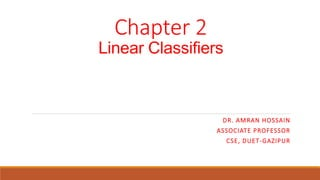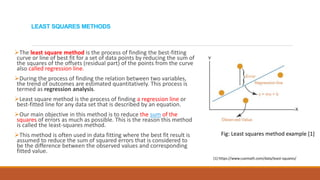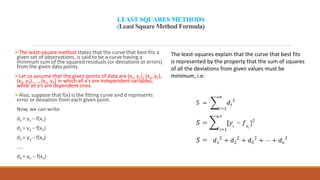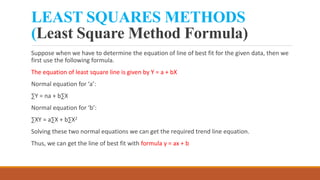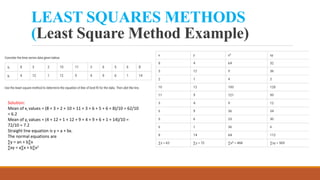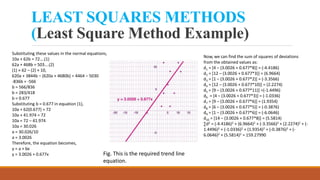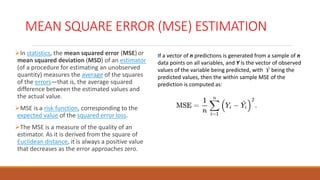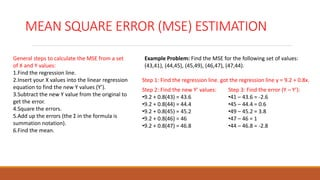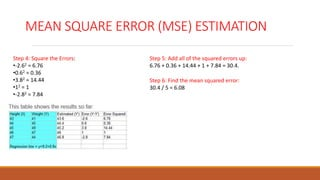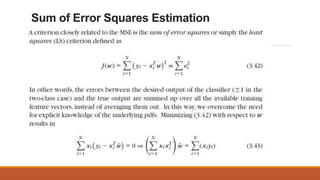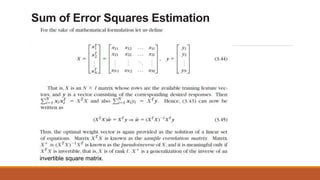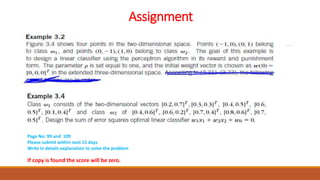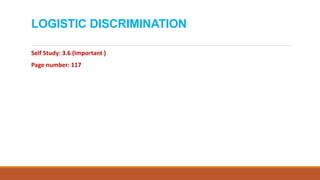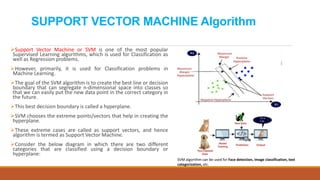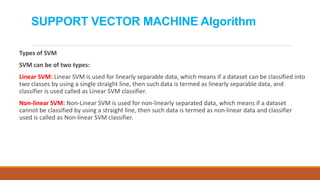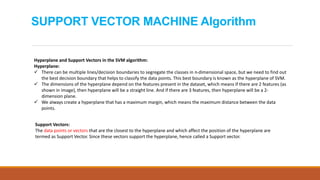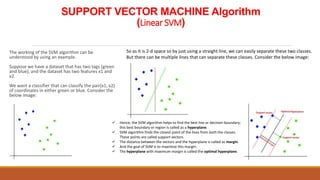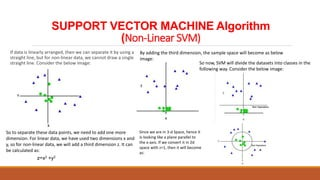1 de 18
Anúncio

### MSE.pptx

1. Chapter 2 Linear Classifiers DR. AMRAN HOSSAIN ASSOCIATE PROFESSOR CSE, DUET-GAZIPUR
2. LEAST SQUARES METHODS The least square method is the process of finding the best-fitting curve or line of best fit for a set of data points by reducing the sum of the squares of the offsets (residual part) of the points from the curve also called regression line. During the process of finding the relation between two variables, the trend of outcomes are estimated quantitatively. This process is termed as regression analysis. Least square method is the process of finding a regression line or best-fitted line for any data set that is described by an equation. Our main objective in this method is to reduce the sum of the squares of errors as much as possible. This is the reason this method is called the least-squares method. This method is often used in data fitting where the best fit result is assumed to reduce the sum of squared errors that is considered to be the difference between the observed values and corresponding fitted value. Fig: Least squares method example   https://www.cuemath.com/data/least-squares/
3. LEAST SQUARES METHODS (Least Square Method Formula) The least-square method states that the curve that best fits a given set of observations, is said to be a curve having a minimum sum of the squared residuals (or deviations or errors) from the given data points. Let us assume that the given points of data are (x1, y1), (x2, y2), (x3, y3), …, (xn, yn) in which all x’s are independent variables, while all y’s are dependent ones. Also, suppose that f(x) is the fitting curve and d represents error or deviation from each given point. Now, we can write: d1 = y1 − f(x1) d2 = y2 − f(x2) d3 = y3 − f(x3) ….. dn = yn – f(xn) The least-squares explain that the curve that best fits is represented by the property that the sum of squares of all the deviations from given values must be minimum, i.e:
4. LEAST SQUARES METHODS (Least Square Method Formula) Suppose when we have to determine the equation of line of best fit for the given data, then we first use the following formula. The equation of least square line is given by Y = a + bX Normal equation for ‘a’: ∑Y = na + b∑X Normal equation for ‘b’: ∑XY = a∑X + b∑X2 Solving these two normal equations we can get the required trend line equation. Thus, we can get the line of best fit with formula y = ax + b
5. LEAST SQUARES METHODS (Least Square Method Example) Solution: Mean of xi values = (8 + 3 + 2 + 10 + 11 + 3 + 6 + 5 + 6 + 8)/10 = 62/10 = 6.2 Mean of yi values = (4 + 12 + 1 + 12 + 9 + 4 + 9 + 6 + 1 + 14)/10 = 72/10 = 7.2 Straight line equation is y = a + bx. The normal equations are ∑y = an + b∑x ∑xy = a∑x + b∑x2
6. LEAST SQUARES METHODS (Least Square Method Example) Substituting these values in the normal equations, 10a + 62b = 72….(1) 62a + 468b = 503….(2) (1) × 62 – (2) × 10, 620a + 3844b – (620a + 4680b) = 4464 – 5030 -836b = -566 b = 566/836 b = 283/418 b = 0.677 Substituting b = 0.677 in equation (1), 10a + 62(0.677) = 72 10a + 41.974 = 72 10a = 72 – 41.974 10a = 30.026 a = 30.026/10 a = 3.0026 Therefore, the equation becomes, y = a + bx y = 3.0026 + 0.677x Fig. This is the required trend line equation. Now, we can find the sum of squares of deviations from the obtained values as: d1 = [4 – (3.0026 + 0.677*8)] = (-4.4186) d2 = [12 – (3.0026 + 0.677*3)] = (6.9664) d3 = [1 – (3.0026 + 0.677*2)] = (-3.3566) d4 = [12 – (3.0026 + 0.677*10)] = (2.2274) d5 = [9 – (3.0026 + 0.677*11)] =(-1.4496) d6 = [4 – (3.0026 + 0.677*3)] = (-1.0336) d7 = [9 – (3.0026 + 0.677*6)] = (1.9354) d8 = [6 – (3.0026 + 0.677*5)] = (-0.3876) d9 = [1 – (3.0026 + 0.677*6)] = (-6.0646) d10 = [14 – (3.0026 + 0.677*8)] = (5.5814) ∑d2 = (-4.4186)2 + (6.9664)2 + (-3.3566)2 + (2.2274)2 + (- 1.4496)2 + (-1.0336)2 + (1.9354)2 + (-0.3876)2 + (- 6.0646)2 + (5.5814)2 = 159.27990
7. MEAN SQUARE ERROR (MSE) ESTIMATION In statistics, the mean squared error (MSE) or mean squared deviation (MSD) of an estimator (of a procedure for estimating an unobserved quantity) measures the average of the squares of the errors—that is, the average squared difference between the estimated values and the actual value. MSE is a risk function, corresponding to the expected value of the squared error loss. The MSE is a measure of the quality of an estimator. As it is derived from the square of Euclidean distance, it is always a positive value that decreases as the error approaches zero. If a vector of n predictions is generated from a sample of n data points on all variables, and Y is the vector of observed values of the variable being predicted, with being the predicted values, then the within sample MSE of the prediction is computed as:
8. MEAN SQUARE ERROR (MSE) ESTIMATION General steps to calculate the MSE from a set of X and Y values: 1.Find the regression line. 2.Insert your X values into the linear regression equation to find the new Y values (Y’). 3.Subtract the new Y value from the original to get the error. 4.Square the errors. 5.Add up the errors (the Σ in the formula is summation notation). 6.Find the mean. Example Problem: Find the MSE for the following set of values: (43,41), (44,45), (45,49), (46,47), (47,44). Step 1: Find the regression line. got the regression line y = 9.2 + 0.8x. Step 2: Find the new Y’ values: •9.2 + 0.8(43) = 43.6 •9.2 + 0.8(44) = 44.4 •9.2 + 0.8(45) = 45.2 •9.2 + 0.8(46) = 46 •9.2 + 0.8(47) = 46.8 Step 3: Find the error (Y – Y’): •41 – 43.6 = -2.6 •45 – 44.4 = 0.6 •49 – 45.2 = 3.8 •47 – 46 = 1 •44 – 46.8 = -2.8
9. MEAN SQUARE ERROR (MSE) ESTIMATION Step 4: Square the Errors: •-2.62 = 6.76 •0.62 = 0.36 •3.82 = 14.44 •12 = 1 •-2.82 = 7.84 Step 5: Add all of the squared errors up: 6.76 + 0.36 + 14.44 + 1 + 7.84 = 30.4. Step 6: Find the mean squared error: 30.4 / 5 = 6.08
10. Sum of Error Squares Estimation
11. Sum of Error Squares Estimation invertible square matrix.
12. Assignment Page No: 99 and 109 Please submit within next 15 days Write in details explanation to solve the problem If copy is found the score will be zero.
13. LOGISTIC DISCRIMINATION Self Study: 3.6 (Important ) Page number: 117
14. SUPPORT VECTOR MACHINE Algorithm Support Vector Machine or SVM is one of the most popular Supervised Learning algorithms, which is used for Classification as well as Regression problems. However, primarily, it is used for Classification problems in Machine Learning. The goal of the SVM algorithm is to create the best line or decision boundary that can segregate n-dimensional space into classes so that we can easily put the new data point in the correct category in the future. This best decision boundary is called a hyperplane. SVM chooses the extreme points/vectors that help in creating the hyperplane. These extreme cases are called as support vectors, and hence algorithm is termed as Support Vector Machine. Consider the below diagram in which there are two different categories that are classified using a decision boundary or hyperplane: SVM algorithm can be used for Face detection, image classification, text categorization, etc.
15. SUPPORT VECTOR MACHINE Algorithm Types of SVM SVM can be of two types: Linear SVM: Linear SVM is used for linearly separable data, which means if a dataset can be classified into two classes by using a single straight line, then such data is termed as linearly separable data, and classifier is used called as Linear SVM classifier. Non-linear SVM: Non-Linear SVM is used for non-linearly separated data, which means if a dataset cannot be classified by using a straight line, then such data is termed as non-linear data and classifier used is called as Non-linear SVM classifier.
16. SUPPORT VECTOR MACHINE Algorithm Hyperplane and Support Vectors in the SVM algorithm: Hyperplane:  There can be multiple lines/decision boundaries to segregate the classes in n-dimensional space, but we need to find out the best decision boundary that helps to classify the data points. This best boundary is known as the hyperplane of SVM.  The dimensions of the hyperplane depend on the features present in the dataset, which means if there are 2 features (as shown in image), then hyperplane will be a straight line. And if there are 3 features, then hyperplane will be a 2- dimension plane.  We always create a hyperplane that has a maximum margin, which means the maximum distance between the data points. Support Vectors: The data points or vectors that are the closest to the hyperplane and which affect the position of the hyperplane are termed as Support Vector. Since these vectors support the hyperplane, hence called a Support vector.
17. SUPPORT VECTOR MACHINE Algorithm (Linear SVM) The working of the SVM algorithm can be understood by using an example. Suppose we have a dataset that has two tags (green and blue), and the dataset has two features x1 and x2. We want a classifier that can classify the pair(x1, x2) of coordinates in either green or blue. Consider the below image: So as it is 2-d space so by just using a straight line, we can easily separate these two classes. But there can be multiple lines that can separate these classes. Consider the below image:  Hence, the SVM algorithm helps to find the best line or decision boundary; this best boundary or region is called as a hyperplane.  SVM algorithm finds the closest point of the lines from both the classes. These points are called support vectors.  The distance between the vectors and the hyperplane is called as margin.  And the goal of SVM is to maximize this margin.  The hyperplane with maximum margin is called the optimal hyperplane.
18. SUPPORT VECTOR MACHINE Algorithm (Non-Linear SVM) If data is linearly arranged, then we can separate it by using a straight line, but for non-linear data, we cannot draw a single straight line. Consider the below image: So to separate these data points, we need to add one more dimension. For linear data, we have used two dimensions x and y, so for non-linear data, we will add a third dimension z. It can be calculated as: z=x2 +y2 By adding the third dimension, the sample space will become as below image: So now, SVM will divide the datasets into classes in the following way. Consider the below image: Since we are in 3-d Space, hence it is looking like a plane parallel to the x-axis. If we convert it in 2d space with z=1, then it will become as:
Anúncio